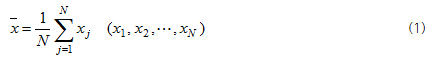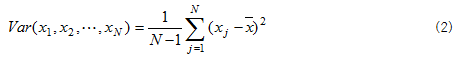Probability Distribution is a method showing the probability distribution of the relevant data value. This analysis additionally shows Mean, Standard Deviation, Skewness, and Kurtosis. These four variables will be explained in detail.

Probability distribution can be characterized by moments. Mean is the first moment value as follow;Mean, also called as expected value, is the value calculated by dividing total sum of signals by the number of signal data. Probability distribution is the one of which sum is 1 to show distribution of probability depending on variables, when values of signals are regarded as variables. Variance, defined as the second moment value, is the value defining the degree of distance from mean in the probability distribution graph as follow;Standard Deviation is a square root of variance as follow;Standard deviation is a value relating to the shape of probability distribution graph. While referring to the following graphs, meaning of these values will be discussed again.

Probability distribution graphs showing mean and standard deviation are presented in the above. In the case of probability distribution in the form of symmetrical mountain as above, the middle value of probability distribution is Mean. Standard Deviation is a value showing the degree that the probability distribution graph is scattered. Lower standard deviation means narrower probability distribution graph, indicating that data are centered on mean. In other words, when standard deviation is low, most data values are similar to mean and differences among data values are not great.
Select data you want to apply this method in Analysis Window and click the icon of Probability Distribution in Analysis Tool Bar. This will display a graph showing analysis results. In the top of probability distribution graph, Mean, Standard Deviation, Skewness, and Kurtosis values are indicated. Analysis results, calculated by channels, are shown as a bar graph, which is registered at Analysis Window.